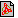Cím: Problems of the Kürschák József mathematical competition Füzet: 1985/február, 80. oldalPDF file Témakör(ök): Kürschák József (korábban Eötvös Loránd)

 A szöveg csak Firefox böngészőben jelenik meg helyesen. Használja a fenti PDF file-ra mutató link-et a letöltésre.1. Writing up the first four rows of the Pascal triangle in the usual way and then adding up the numbers standing under one another, we obtain the following seven numbers: $1$, $1$, $4$, $3$, $4$, $1$, $1$.If we write up the first 1024 rows of the Pascal triangle and then add up the numbers standing under one another, how many of the 2047 numbers thus obtained will be odd ? 2. ${A}_{1}{B}_{1}{A}_{2}$,  ${B}_{1}{A}_{2}{B}_{2}$,  ${A}_{2}{B}_{2}{A}_{3}$,  $\text{...}$,  ${B}_{13}{A}_{14}{B}_{14}$,  ${A}_{14}{B}_{14}{A}_{1}$,  ${B}_{14}{A}_{1}{B}_{1}$ are adjacent rigid regular plates which can be folded along the edges ${A}_{1}{B}_{1}$,  ${B}_{1}{A}_{2}$,  $\text{...}$,  ${A}_{14}{B}_{14}$,  ${B}_{14}{A}_{1}$. Can they be folded so that all the 28 plates be in the same plane? 3. Given are $n$ not necessarily different integers and two positive integers $p$ and $q$. Choose two equal ones of the $n$ numbers, add $p$ to one of them and subtract $q$ from the other one. If there are still equal ones among the $n$ numbers then repeat this procedure. Prove that after a finite number of steps one arrives at $n$ different numbers.